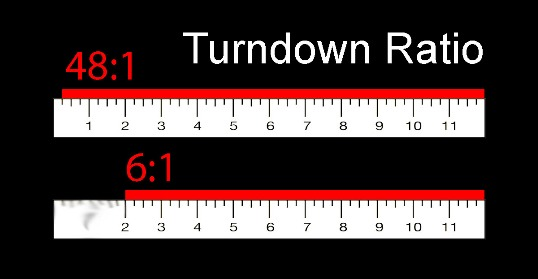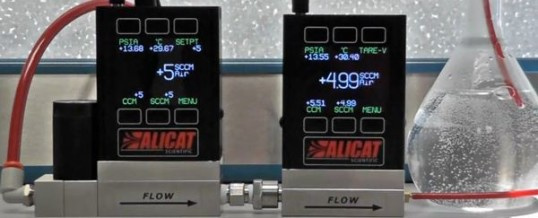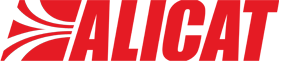# The Significance of Turndown Ratio for Mass Flow Controllers

Turndown ratio can be defined as a measure of the usable range of an instrument. It is expressed as the full-scale range divided by the lowest point of measure. It indicates the instrument range required to generate precise readings, which is crucial when measuring or controlling a very broad flow range without modifying the instruments.

## Calculation of Turndown Ratio

Take a 12" ruler, which is graduated to a quarter of an inch. The smallest distance measurable is 1/4", and the turndown ratio is 12"/0.25" = 48 to 1 (48:1). In case, the same ruler has smudged the graduated lines below 2", the precision still remains the same as the distance between marks is the same; however, the usable range is less. Here the turndown ratio is 12"/2.0" = 6:1. Using this smudged ruler, an object measuring about an inch and a half in length cannot be precisely measured. To achieve this, a ruler that is smaller with the same turndown would be required, about 6" with lines smudged below 1"(Figure 1). Another option would be to use the same size ruler with a larger turndown ratio of about 12:1.Figure 1. Turndown ratio

A flow controller or flow meter’s turndown ratio is highly influenced by the device’s signal-to-noise ratio, which is a result of the type and quality of the sensor used, and the fluid dynamics of the flow measurement technology. For instance, many orifice-plate differential pressure-based flow measurement devices possess turndown ratios of 4:1, which points out that precision is not properly maintained below 25% of the full-scale flow.

Based on Bernoulli’s Principle, differential pressure is correlated to the square of the flow velocity within turbulent (non-laminar) flows. Therefore, decreasing the full-scale flow rate by two minimizes the differential pressure by four, and decreasing the full-scale flow rate by four minimizes the differential pressure by 16. Smaller differential pressures would mean smaller sensor signal strength, and ultimately lost signals in the signal noise.

In comparison, laminar differential pressure-based flow measurement devices have a direct linear relationship between flow velocity and differential pressure. According to Poiseuille’s Equation, this is achieved by moving the turbulent flow into laminar channels, where flow velocity and differential pressure are linearly linked. So in an Alicat laminar mass flow instrument decreasing the full-scale flow rate by four only minimizes the differential pressure by four. This, along with Alicat’s sensor technology, ensures precision in several of the company’s mass flow products to at least 0.5% of the full-scale flow rate, a turndown ratio of 200:1 (Figure 2).Figure 2. Alicat’s mass flow products have accuracy of at least 0.5% of the full-scale flow rate, a turndown ratio of 200:1.

## Alicat’s Turndown Test

The video below illustrates the results of Alicat’s latest turndown ratio test. In this test an unedited, real-time video is used to observe the actual flow rate from a 5-slpm mass flow controller. However, Alicat did not pair this controller with a 5-slpm meter, instead it was paired with a 50-sccm mass flow meter to observe what the lowest its controller could achieve was. At full scale, the range of this meter specifies just the bottom 1% of the flow controller’s range, a 100:1 turndown. The test is begun at a flow rate of 50 sccm, which specifies 100:1 turndown for the controller, and subsequently proceeds to 5 sccm (1000:1) and 1 sccm (5000:1).

All of the monitored flow rates shown in the video were controlled by the mass flow controller to within 0.13 sccm, which is an outstanding accomplishment for a 5000-sccm controller.

## Conclusion

If a flow controller with a 50:1 turndown ratio is used, Alicat recommends using one device capable of 100-5000 sccm, another one to capable of 2-100 sccm, and a third one capable of 1-2 sccm. Using higher turndown ratios means spending less money to monitor wide flow ranges. Higher turndown ratios facilitate better flexibility when an experiment suddenly needs a wider range. A high turndown ratio can be an asset to a project when it is necessary to go lower than planned.This information has been sourced, reviewed and adapted from materials provided by Alicat Scientific.

## Citations

• APA

Alicat Scientific, Inc.. (2019, May 03). The Significance of Turndown Ratio for Mass Flow Controllers. AZoM. Retrieved on October 24, 2020 from https://www.azom.com/article.aspx?ArticleID=12616.

• MLA

Alicat Scientific, Inc.. "The Significance of Turndown Ratio for Mass Flow Controllers". AZoM. 24 October 2020. <https://www.azom.com/article.aspx?ArticleID=12616>.

• Chicago

Alicat Scientific, Inc.. "The Significance of Turndown Ratio for Mass Flow Controllers". AZoM. https://www.azom.com/article.aspx?ArticleID=12616. (accessed October 24, 2020).

• Harvard

Alicat Scientific, Inc.. 2019. The Significance of Turndown Ratio for Mass Flow Controllers. AZoM, viewed 24 October 2020, https://www.azom.com/article.aspx?ArticleID=12616.